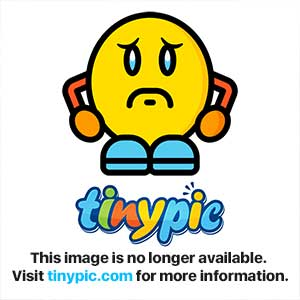# Preview/Image point/Colision Mask windows frozen (Linux)

Hallo ^^

I got recently Game Develop (Ubuntu version) and it works (mostly) perfectly here. The major problem is on Sprite Editor. Clicking on Preview, Collision Mask Editor or Center view, these windows appear, but frozen: I can’t edit the attributes nor close them. Closing the Sprite Editor, however, close the frozen window together.

I hope the Game Develop’s team could solve this problem soon, the tool has a good potential, not only on Linux, but also on Windows.

My congratulations for the team as well, by creating such great program, accessible for everyone.

There are some images which may explain it better:• Opening a random game on Game Develop (In this case, “Lazer and Zombies”)…• I open the Sprite (Object) Editor…• And here’s the problem: I open, for example, the Collision Mask Editor. The window open, but I can’t edit, move or even close it, as well edit the configurations…• The window is frozen, however, the rest of the program is still working. Closing the Object Editor will close the frozen window too.

I’m not sure if the problem happends because I’m using XCFE, in any case, I hope the team can fix this problem.

serius bug , i want create a game and has presented in linux, the mask or center can’t editin this period i not use game develop with sprite , 4ian the linux version is unusable, please fix it
the error

code: Gtk-CRITICAL **: IA__gtk_widget_get_direction: assertion `GTK_IS_WIDGET (widget)’ failed

(GDIDE:3830): Gtk-CRITICAL **: IA__gtk_window_resize: assertion `width > 0’ failed
[/code]

how can I do this?

is It in preferences? an indication is appreciate

Try to do this :

-Close Game Develop
-Go to your home directory, then in “.Game Develop” folder and open “options.cfg”
-Search for the [SpriteObjectEditor] section in this file.
-Replace the content of the section with this :

```[SpriteObjectEditor] LastWorkspace=layout2|name=centerPane;caption=Pane caption;state=2229244;dir=5;layer=0;row=0;pos=0;prop=100000;bestw=124;besth=70;minw=-1;minh=-1;maxw=-1;maxh=-1;floatx=-1;floaty=-1;floatw=-1;floath=-1|name=animationsPane;caption=Animations;state=2044;dir=4;layer=0;row=0;pos=0;prop=100000;bestw=200;besth=105;minw=200;minh=75;maxw=-1;maxh=-1;floatx=-1;floaty=-1;floatw=-1;floath=-1|name=imagePane;caption=Images;state=2044;dir=3;layer=0;row=0;pos=0;prop=100000;bestw=100;besth=80;minw=400;minh=150;maxw=-1;maxh=-1;floatx=-1;floaty=-1;floatw=-1;floath=-1|name=maskPane;caption=Collision masks;state=2099198;dir=4;layer=0;row=0;pos=1;prop=100000;bestw=166;besth=45;minw=200;minh=150;maxw=-1;maxh=-1;floatx=-1;floaty=415;floatw=400;floath=250|name=pointsPane;caption=Points;state=2099196;dir=4;layer=0;row=0;pos=1;prop=100000;bestw=306;besth=181;minw=200;minh=150;maxw=-1;maxh=-1;floatx=-1;floaty=-1;floatw=-1;floath=250|name=previewPane;caption=Preview;state=2099198;dir=2;layer=0;row=0;pos=1;prop=100000;bestw=20;besth=20;minw=150;minh=150;maxw=-1;maxh=-1;floatx=505;floaty=437;floatw=400;floath=250|name=ResourcesEditor;caption=Resources editor;state=4196348;dir=2;layer=0;row=0;pos=0;prop=100000;bestw=230;besth=100;minw=200;minh=150;maxw=-1;maxh=-1;floatx=-1;floaty=-1;floatw=-1;floath=-1|dock_size(5,0,0)=126|dock_size(4,0,0)=202|dock_size(3,0,0)=169|dock_size(2,0,0)=202|```

-Close the file, launch Game Develop. Now points and collisions masks window should be docked and usable.

nothing to do,
i have noted one thing, the [SpriteObjectEditor] not is created, anyway i add lines at the end of file, the dimension of pannel is modified , but same error